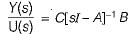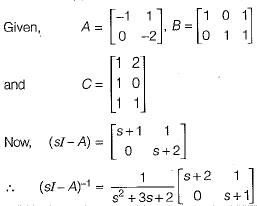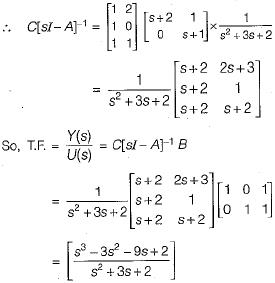Courses

# State Variable Analysis

## 10 Questions MCQ Test Mock Tests Electronics & Communication Engineering GATE 2020 | State Variable Analysis

Description
This mock test of State Variable Analysis for GATE helps you for every GATE entrance exam. This contains 10 Multiple Choice Questions for GATE State Variable Analysis (mcq) to study with solutions a complete question bank. The solved questions answers in this State Variable Analysis quiz give you a good mix of easy questions and tough questions. GATE students definitely take this State Variable Analysis exercise for a better result in the exam. You can find other State Variable Analysis extra questions, long questions & short questions for GATE on EduRev as well by searching above.
QUESTION: 1

Solution:
QUESTION: 2

Solution:
QUESTION: 3

### For a linear time invariant system, an optimal controller can be designed if

Solution:

An optimal controller for an LTI system can be designed provided the system is both controllable and observable

QUESTION: 4

The eigen values of linear system are the location of

Solution:

Eigen value are given by | sI- A I = 0, which is the location of poles.

QUESTION: 5

If the system matrix of a linear time invariant continuous system is given by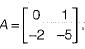its characteristic equation will be given by

Solution:

Characteristic equation is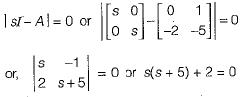or, s2 + 5s + 2 = 0

QUESTION: 6

​The state-variable description of a linear autonomous system is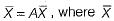is a two-dimensional state vector and A is a matrix given by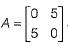The poles of the system are located at

Solution:

Poles of given system are given by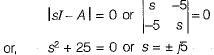QUESTION: 7

Assertion (A): The eigen values of a linear continuous-data time invariant system controls the stability of the system.
Reason (R): The roots of the characteristic equation are the same as the eigen values of system matrix A of the state equations.

Solution:
QUESTION: 8

The vector matrix differential equation of a system is given by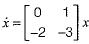The state transition matrix of the system is

Solution:

We have: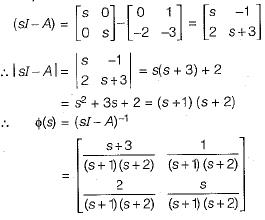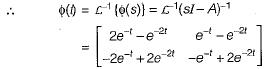QUESTION: 9

The system matrix of a continous time system is given by: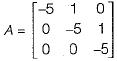​The characteristic equation is

Solution:

The characteristic equation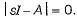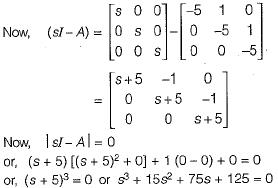QUESTION: 10

A system is described by the following equations: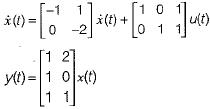The transfer function of the system is

Solution:

Transfer function is given by